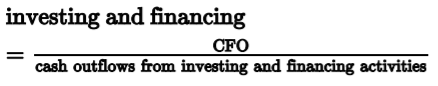# Calculate free cash flow

• Represents the cash a company generates after cash outflows to support operations and maintain its capital assets.
• Available once firm covered capital expenditures
• Often used for valuation
• Two main measures of free cash flow
• Free cash flow to the firm (FCTF) (4)
• Free to all investors: debt holders + equity owners
• Calculate from net income
•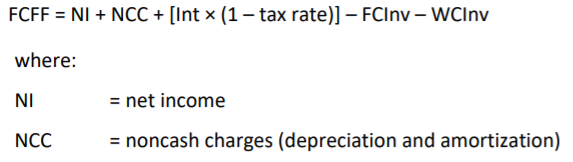•• Calculate from CFO (5)
•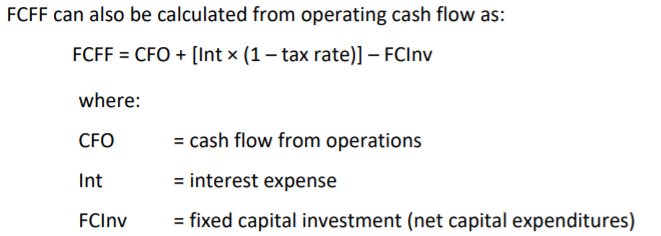• Change in working capital and non-cash charge is already included in CFO
• Dividends paid would be added back to CFO if firms follow IFRS

Calculate free cash flow to Equity

• Free Cash Flow to Equity (6)
• CF that would be available for distribution to common shareholders

Calculate free cash flow to Equity

• Other CF Ratios (7)
• Performance Ratios:
•  Cash flow to revenue (8): amount of CFO generated for each dollar of revenue
•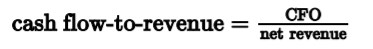• Return on assets (9)
•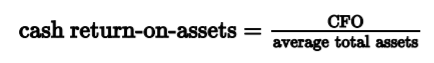• Return on equity ratio (10): return of CFO attributed to shareholder
•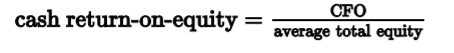• Cash-to-income (11): ability to generate cash from operation
•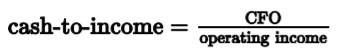• Cash flow per share (12): variation of basic earnings per share
•• Converge ratio (8)
• Debt coverage ratio (13): financial risk and leverage
•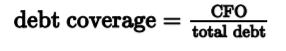• Interest coverage ratio (14): ability to meet interest obligation
•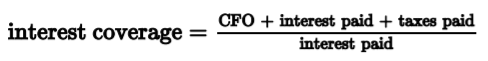• Reinvestment ratio (15): ability to acquire long term assets
•• Debt payment ratio(16): ability to satisfy long-term debt
•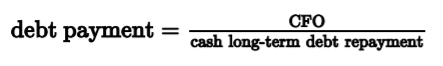• Dividend payment ratio(17): ability to make dividend payment from CFO
•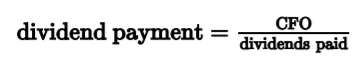• Investing and financing ratio(18): ability to purchase assets, satisfy debts and pay dividends
•